×

×

UA / Economics / STAT 309 / the index of leading indicators compiled by the conference board inclu

# the index of leading indicators compiled by the conference board inclu Description

##### Description: This study guide covers chapter 10-14. Each chapter contains notes as well as 10 possible test questions.
14 Pages 6 Views 9 Unlocks
Reviews

EC 309

## What s Okun's law?EC 309 Intermediate Macroeconomics Test 2 Study Guide

Chapter 10: Introduction to Economic Fluctuations ∙ Okun’s law- the negative relationship between GDP and unemployment ∙ Leading indicators- variables that tend to fluctuate in advance of the overall  economy

o Published monthly by the Conference Board

o Aims to forecast changes in economic activity 6-9 months into the future o Used in planning by businesses and government, despite not being a perfect  predictor

∙ Time horizons:

o Long run- prices are flexible, respond to changes in supply or demand o Short run- many prices are “sticky” at a predetermined level

∙ Aggregate demand- the relationship between the quantity of output demanded  and the aggregate price level

o MV=PY

*** an increase in the money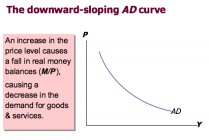## What is demand shocks?supply shifts the AD curve to If you want to learn more check out which measure of central tendency can be used for both numerical and categorical variables

the right

o

∙ Aggregate supply- the relationship between the quantity of goods and services  supplied and the price level

o Y= F (K, L)

*** an increase in M shifts increases price

 output remains the same

o

EC 309

Hoda Karasky o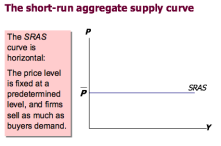## What is Monetary transmission*** an increase in AD  causes output to rise

∙ Shocks- exogenous changes in aggregate supply or demand

o Shocks temporarily push the economy away from full employment ∙ Demand shocks- a shock that shifts the AD curve We also discuss several other topics like bio 303 ub

o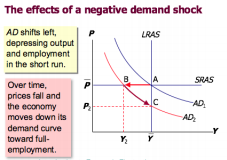We also discuss several other topics like which type of tissue conducts electrical impulses

∙ Supply shocks- a shock that shifts the AS curve

o alters production costs, affects the prices that firms charge

∙ Stabilization policy- policy actions aimed at reducing the severity of short-run  economic fluctuations

o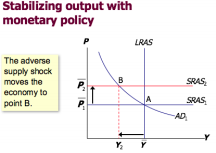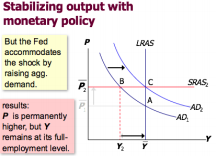Practice Questions for Chapter 10:

1. When GDP growth declines, investment spending typically _______ and  consumption spending typically _______.

a. Increases; increases

b. Increases; decreases

c. Decreases; decreases

d. Decreases; increases

EC 309

2. Okun’s law is the ________ relationship between real GDP and the ________. a. Negative; unemployment rate

b. Negative; inflation rate We also discuss several other topics like econ exam 3

c. Positive; unemployment rate

d. Positive; inflation rate If you want to learn more check out mgs 3100 gsu

3. Long-run growth in real GDP is determined primarily by _________, while short-run  movements in real GDP are associated with ________.

a. Variations in labor market utilization; technological progress Don't forget about the age old question of solvency ratios measure the short-term ability of the company to pay its maturing obligations.

b. Technological progress; variations in labor market utilization

c. Money supply growth rates; changes in velocity

d. Changes in velocity; money supply growth rates

a. The most popular economic statistics

b. Data that are used to construct the consumer price index and the  unemployment rate

c. Variables that tend to fluctuate in advance of the overall economy d. Standardized statistics compiled by the National Bureau of Economic  Research

5. Most economists believe that prices are:

a. Flexible in the short run but many are sticky in the long run

b. Flexible in the long run but many are sticky in the short run

c. Sticky in both the short run and long run

d. Flexible in both the short run and long run

6. A 5% reduction in the money supply will, according to most economists, reduce  price 5%:

a. In both the short run and long runs

b. In neither the short nor long run

c. In the short run but lead to unemployment in the long run

d. In the long run but lead to unemployment in the short run

7. Monetary neutrality, the irrelevance of the money supply in determining values of  _________ variables, is generally thought to be a property of the economy in the  long run.

a. Real

b. Nominal

c. Real and nominal

d. Neither real nor nominal

8. When the Federal Reserve reduces the money supply, at a given price level the  amount of output demanded is ________ and the aggregate demand curve shifts  __________.

a. Greater; inward

b. Greater; outward

c. Lower; inward

d. Lower; outward

9. If the short run aggregate supply curve is horizontal and the long run aggregate  supply curve is vertical, then a change in the money supply will change ________ in  the short run and change _______ in the long run.

a. Only prices; only output

b. Only output; only prices

c. Both prices and output; only prices

d. Both prices and output; both prices and output

EC 309

10. An adverse supply shock ______ the short run aggregate supply curve ______  the natural level of output.

a. Raises; but cannot affect

b. Raises; and may also lower

c. Lowers; but cannot affect

d. Lowers; and may also lower

Chapter 11: Aggregate Demand I: Building the IS-LM Model ∙ IS-LM model- the model of aggregate demand

∙ IS curve- investment and savings; goods and services market

∙ LM curve- liquidity and money; money market

∙ Keynesian cross

o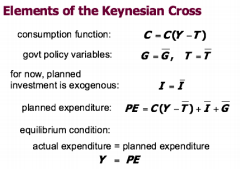o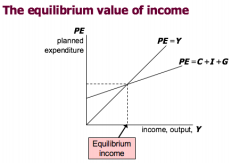∙ Government purchases multiplier

o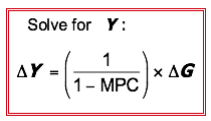EC 309

∙ Tax multiplier

o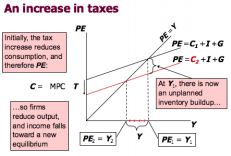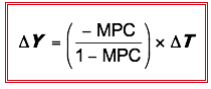∙ Theory of liquidity preferences- the interest rate is determined by money  supply and money demand

Practice Questions for Chapter 11:

1. The IS-LM model takes _________ as exogenous.

a. The price level and national income

b. The price level

c. National income

d. The interest rate

2. In the Keynesian cross model, if the MPC equals 0.75, then a \$1 billion increase in  government spending increases planned expenditures by _______ and increases the equilibrium level of income by ________.

a. \$1 billion; more than \$1 billion

b. \$0.75 billion; more than \$0.75 billion

c. \$0.75 billion; \$0.75 billion

d. \$1 billion; \$1 billion

3. Tax cuts stimulate _________ by improving workers’ incentive and expand __________ by raising households’ disposable income.

a. Velocity; demand for loanable funds

b. Demand for loanable funds; velocity

c. Aggregate demand; aggregate supply

d. Aggregate supply; aggregate demand

4. In the Keynesian cross model, a decrease in the interest rate ___________ planned  investment spending and ___________ the equilibrium level of income. a. Increases; increases

b. Increases; decreases

c. Decreases; decreases

d. Decreases; increases

5. Changes in fiscal policy shift the:

a. LM curve

b. Money demand curve

c. Money supply curve

d. IS curve

6. According to the theory of liquidity preference, if the demand for real money  balances exceeds the supply of real money balances, individuals will:  a. Sell interest earning assets in order to obtain non interest bearing money

EC 309

b. Purchase interest earning assets in order to reduce holdings of non interest  bearing money

c. Purchase fewer goods and services

d. Be content with their portfolios

7. An increase in income raises money ________ and _________ the equilibrium interest  rate.

a. Demand; raises

b. Demand; lowers

c. Supply; raises

d. Supply; lowers

8. Equilibrium levels of income and interest rates are ________ related in the goods  and services market, and equilibrium levels of income and interest rates are  _______ related in the market for real money balances.

a. Positively; positively

b. Positively; negatively

c. Negatively; negatively

d. Negatively; positively

9. In the Keynesian cross analysis, if the consumption function is given by C= 100+  0.6(Y-T), and planned investment is 100, G is 100, and T is 100, then equilibrium Y  is:

a. 350

b. 400

c. 600

d. 750

10. Assume that the money demanded function is (M/P)d = 2,200 – 200r, where r is the interest rate in percent. The money supply M is 2,000 and the price level P is  2. If the price level is fixed and the Fed wants to fix the interest rate at 7 percent,  it should set the money supply at:

a. 2,000

b. 1,800

c. 1,600

d. 1,400

Chapter 12: Aggregate Demand II: Applying the IS-LM  Model

∙ Equilibrium in the IS-LM model:

The intersection determines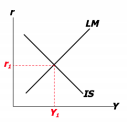the unique combination of Y

and r

that satisfies equilibrium in

o

o Uses of the IS-LM model:

 fiscal policy: G and/or T

 monetary policy: M

EC 309

o Fiscal:

o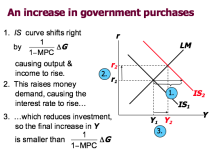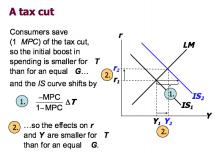o Monetary:

o

∙ Monetary transmission- an increase in the money supply lowers the interest  rate, which stimulates investment and thereby expands the demand for goods and  services

∙ IS shocks- exogenous changes in the demand for goods and services ∙ LM shocks- exogenous changes in the demand for money

∙ Pigou effect- the increase in consumer spending that results when a fall in the  price level raises real money balances and, thereby, consumers’ wealth ∙ Debt-deflation theory- a theory according to which an unexpected fall in the  price level redistributes real wealth from debtors to creditors and, therefore,  reduces total spending in the economy

Practice Questions for Chapter 12:

1. In the IS-LM model, a decrease in government purchases leads to a(n) ________ in  planned expenditures, a(n) ________ in total income, a(n) _________ in money  demand, and a(n) __________ in the equilibrium interest rate.

a. Decrease; decrease; decrease; decrease

b. Increase; increase; increase; increase

c. Decrease; decrease; increase; increase

d. Increase; increase; decrease; decrease

2. In the IS-LM model under the usual conditions in a closed economy, an increase in  government spending increases the interest rate and crowds out:

EC 309

a. Prices

b. Investment

c. The money supply

d. Taxes

3. In the IS-LM model when M/P rises, in short run equilibrium, in the usual case the  interest rate ________ and output __________.

a. Rises; falls

b. Rises; rises

c. Falls; rises

d. Falls; falls

4. If taxes are raised, but the Fed prevents income from falling by raising the money  supply, then:

a. Both consumption and investment remain unchanged

b. Consumption rises but investment falls

c. Investment rises but consumption falls

d. Both consumption and investment fall

5. An increase in the demand for money, at any given income level and level of  interest rates, will, within the IS-LM framework, ______ output and ________ interest  rates.

a. Increase; lower

b. Increase; raise

c. Lower; lower

d. Lower; raise

6. The aggregate demand curve generally slopes downward and to the right because, for any given money supply M a high price liven P causes a _________ real money  supply M/P, which _________ the interest rate and _________ spending. a. Lower; raises; reduces

b. Higher; lowers; increases

c. Lower; lowers; increases

d. Higher; raises; reduces

7. A decrease in the price level shifts the ________ curve to the right, and the  aggregate demand curve _________.

a. IS, shifts to the right

b. IS, does not shift

c. LM, shifts to the right

d. LM, does not shift

8. Analysis of the short and long runs indicates that the ________ assumptions are  most appropriate in ____________.

a. Classical; both the short and long runs

b. Keynesian; both the short and long runs

c. Classical; the short run, whereas the Keynesian assumptions are most  appropriate in the long run

d. Keynesian; the short run, whereas the classical assumptions are most  appropriate in the long run

9. The Pigou effect suggests that falling prices will increase income because real  balances influence _______ and will shift the ____ curve.

a. Money demand; LM

b. The money supply; LM

c. Consumer spending; IS

d. Government spending; IS

EC 309

10. If consumption is given by C= 200+ 0.75(Y-T) and investment is given by I=  200- 25r, then the formula for the IS curve is:

a. Y= 400 – 0.75T -25r +G

b. Y= 1,600 – 3T -11r +4G

c. Y= 400+ 0.75T- 25r – G

d. Y= 1,600 +3T – 100r - 4G

Chapter 13: The Open Economy Revisited: The Mundell  Fleming Model

∙ Mundell Fleming model

o  ∙ IS curve:

↓ ⇒ ↑ ⇒ ↑

o

∙ Floating exchange rates- e is allowed to fluctuate in response to changing  economic conditions

∙ Fixed exchange rates- the central bank trades domestic for foreign currency at a predetermined price

∙ Fiscal Floating Monetary  Floating o  ∙ Trade  Floating

EC 309

o

∙ Fiscal  Fixed Monetary  Fixed o  ∙ Trade  Fixed

o

∙ Country risk- the risk that the country’s borrowers will default on their loan  repayments because of political or economic turmoil; lenders require a higher  interest rate to compensate them for this risk

∙ Expected exchange rate changes- if a country’s exchange rate is expected to  fall, then its borrowers must pay a higher interest rate to compensate lenders for  the expected currency depreciation

Practice Questions for Chapter 13:

1. In the Mundell Fleming model, the domestic interest rate is determined by the: a. Intersection of the LM and IS curves

b. Domestic rate of inflation

c. World rate of inflation

d. World interest rate

2. In a small open economy with a floating exchange rate, the exchange rate will  appreciate if:

a. The money supply is increased

b. The money supply is decreased

EC 309

c. Government spending is decreased

d. Taxes are increased

3. In a small open economy with a floating exchange rate, the supply of real money  balances is fixed and a rise in government spending:

a. Raises the interest rate, so that income must rise to maintain equilibrium in  the money market

b. Raises the interest rate so that net exports must fall to maintain equilibrium  in the goods market

c. Cannot change the interest rate so that net exports must fall to maintain  equilibrium in the goods market

d. Cannot change the interest rate so income must rise to maintain equilibrium  in the money market

4. To maintain a fixed exchange rate system, if the exchange rate moves below the  fixed exchange rate level, then the central bank must:

b. Sell foreign currency from reserves

c. Raise taxes

d. Decrease government spending

5. In a small open economy with a fixed exchange rate, an effective policy to increase equilibrium output is to:

a. Decrease government spending

b. Decrease taxes

c. Increase the money supply

d. Decrease the money supply

6. In the Mundell Fleming model with fixed exchange rates, attempts by the central  bank to decrease the money supply:

a. Lead to a lower equilibrium level of income

b. Lead to a higher equilibrium level of income

c. Must be abandoned in order to maintain the fixed exchange rate d. Must be offset by expansionary fiscal policy

7. The risk premium included in the interest rate of small open economies  incorporates:

a. Country risk and expectations of future exchange rate changes

b. The law of one price

c. Inefficient activity by arbitrageurs

d. Capital mobility

8. The “impossible trinity” refers to the idea that it is impossible for a country to  simultaneously have:

a. Low inflation, low unemployment, and rapid rate of GDP growth

b. Free capital flows, a fixed exchange rate, and an independent monetary  policy

c. High interest rates, a budget deficit, and a trade deficit

d. An expansionary fiscal policy, a contractionary monetary policy, and a  flexible exchange rate

9. In the Mundell Fleming model, if the price level falls, then the equilibrium income a. Rises and the real exchange rate appreciates

b. Rises and the real exchange rate depreciates

c. Falls and the real exchange rate appreciates

d. Falls and the real exchange rate depreciates

EC 309

10. A fall in consumer confidence about the future, which induces consumers to  spend less and save more, will, according to the Mundell Fleming model with  floating exchange rates, lead to:

a. A fall in consumption and income

b. No change in consumption or income

c. No change in income but a rise in net exports

d. No change in income or net exports

Chapter 14: Aggregate Supply and the Short-run Tradeoff  Between Inflation and Unemployment

∙ Sticky price model:

o long-term contracts between firms and customers

o firms not wishing to annoy customers with frequent price changes o p P = + a ( ) Y − Y

o

∙ Imperfect information model:

o All wages and prices are perfectly flexible; all markets are clear. o Each supplier produces one good, consumes many goods.

o Each supplier knows the nominal price of the good she produces, but does  not know the overall price level

∙ Phillip’s curve- states that inflation depends on:

EC 309

o expected inflation, Eπ.

o cyclical unemployment: the deviation of the actual rate of unemployment from the natural rate

o supply shocks

= E u − − u +

( )n

o

∙ Adaptive expectations- an approach that assumes people form their  expectations of future inflation based on recently observed inflation ∙ Cost-push inflation- inflation resulting from supply shocks

o Adverse supply shocks typically raise production costs and induce firms to  raise prices,

“pushing” inflation up.

∙ Demand-pull inflation- inflation resulting from demand shocks o Positive shocks to aggregate demand cause unemployment to fall below its  natural rate,

which “pulls” the inflation rate up

∙ Sacrifice ratio- measures the percentage of a year’s real GDP that must be  foregone to reduce inflation

by 1 percentage point

∙ Rational expectations- people base their expectations on all available  information, including information about current and prospective future policies

Practice Questions for Chapter 14:

1. According to the sticky price model, other things being equal, the greater the  proportion, s, of firms that follow the sticky price rule, the ______ the ______ in  output in response to an unexpected price increase.

a. Greater; increase

b. Smaller; increase

c. Greater; decrease

d. Greater; increase

2. The imperfect information model bases the difference in the short run and long run aggregate supply curve on:

a. Sticky wages

b. Sticky prices

EC 309

d. Procyclical real wages

3. Both models of aggregate supply discussed in Chapter 14 imply that if the price  level is higher than expected, then output _______ natural rate of output.  a. Exceeds the

b. Falls below the

c. Equals the

d. Moves to a different

4. In the short run, if the price level is greater than the expected price level, then in  the long run the aggregate:

a. Demand curve will shift leftward

b. Demand curve will shift rightward

c. Supply curve will shift upward

d. Supply curve will shift downward

5. The Phillips curve depends on all of the following forces except

a. The current exchange rate

b. Expected inflation

c. The deviation of unemployment from its natural rate

d. Supply shocks

6. The classical dichotomy breaks down for a Phillips curve, which shows the  relationship between a nominal variable, _______, and a real variable _________. a. Output; prices

b. Money; output

c. Inflation; unemployment

d. Unemployment; inflation

7. Inflation inertia is represented in the aggregate supply- aggregate demand model  by continuing upward shifts in the:

a. Aggregate demand curve

b. Short run aggregate supply curve

c. Long run aggregate supply curve

d. Aggregate demand and short run aggregate supply curves

8. In the case of demand-pull inflation, other things being equal:

a. Both the inflation rate and the unemployment rate rise at the same time b. The unemployment rate rises but the inflation rate falls

c. The inflation rate rises but the unemployment rate falls

d. Both the inflation rate and the unemployment rate fall

9. The government can lower inflation with a low sacrifice ratio if the: a. Money supply is reduced slowly

c. Short run aggregate supply schedule is relatively flat

d. Public believes that policymakers are committed to reducing inflation 10. Assume that an economy has the usual type of Phillips curve except that the  natural rate of unemployment in an economy is given by an average of the  unemployment rates in the last two years. Then, there is:

a. A long run tradeoff between inflation and unemployment

b. No long run tradeoff between inflation and unemployment

c. No short run tradeoff between inflation and unemployment

d. A sacrifice ratio that is large but not infinite

Page ExpiredIt looks like your free minutes have expired! Lucky for you we have all the content you need, just sign up here
References: